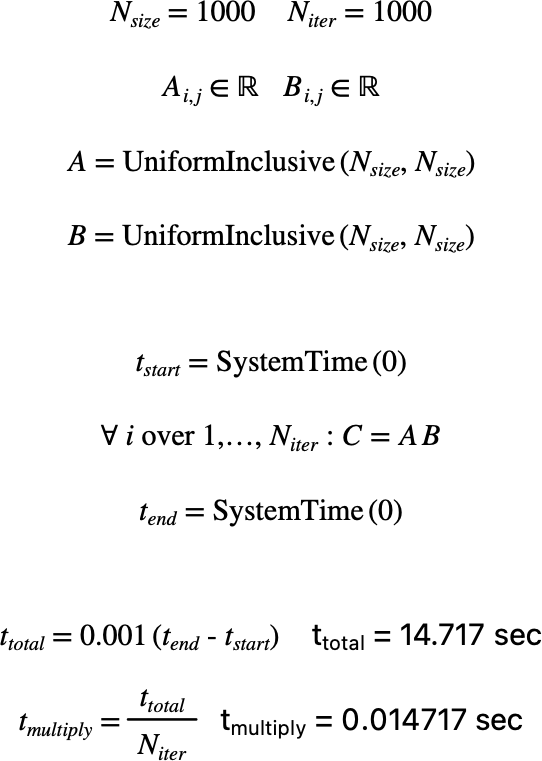# $$\text{SystemTime}$$¶

You can use the $$\text{SystemTime}$$ function to obtain the current system time in mSec since midnight January 1, 1970, UTC.

You can use the \systemtime backslash command to insert this function.

The following variants of this function are available:

• $$\text{integer } \text{SystemTime} \left ( \text{<any value>} \right )$$

The $$\text{SystemTime}$$ function requires a single arbitrary parameter that is ignored. The function returns an integer value indicating the number of milliseconds that have elapsed since midnight, January 1, 1970 UTC.

The $$\text{SystemTime}$$ function is primarily intended to allow you to measure elapsed time for benchmarking purposes.

Figure 209 shows the basic use of the $$\text{SystemTime}$$ function.Figure 209 Example Use Of The SystemTime Function